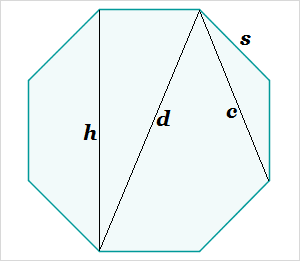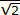# Octagon Measurement Calculator

Octagon Measurement Calculator
s =
h =
d =
c =
area =
perimeter =
Select the radio button for one of the dimensions, enter its value, and click the calculate button to obtain the other measurementsThe side length, height, diagonals, and area of a regular octagon are all interrelated. If you know the measure of one dimension, you can calculate the others. For example, if the side length is s, the height h, the main diagonal d, the minor diagonal c, the area a, and the perimeter p, then you can calculate the following from s:

h = (1+√2)s

d = (√4+2)s

c = (√2+)s

a = (2+2√2)s²

p = 8s

Similarly,

s = h/(1+√2) = 0.5(√2 - 1)h

d = 0.5(√2+)h, etc.

You can perform these computations using a hand calculator, our online scientific calculator, or the convenient octagonal measurement calculator at left. All results are accurate within 5 decimal places.